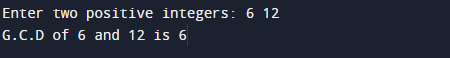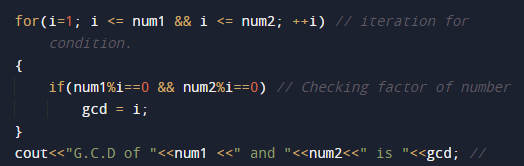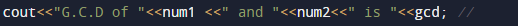C++ Program to Find GCD

In this tutorial you will learn about the C++ Program to Find GCD and its application with practical example.

In this tutorial, we will learn to create a C++ program that  will find GCD or HCF of two number  using C++ programming.

Prerequisites

Before starting with this tutorial we assume that you are best aware of the following C  ++ programming topics:

• Operators.
• General C programming.
• Basic input/output.
• Looping statements
• While loop.
• if else Statement.

What Is GCD?

GCD(Greatest Common Divisor) or HCF (Highest Common Factor) . When  we finding the factor of two numbers, Highest Common Factor of  numbers is the Largest factor that divides these two numbers. The greatest factor found from the common factors is called the HCF. It is also called the Greatest common factor.

C++ Program to Find GCD.

In this program we will find GCD of two given number using For loop. We would first declared and initialized the required variables. Next, we would prompt user to input two numbers.Later we will find the GCD of given number.

OutputIn the above program, we have first declared and initialized a set variables required in the program.

• num1 =It will hold first number given by user.
• num2 =It will hold second number given by user.
• gcd= it will hold Greatest common divisor.

Let see the step by step method of finding GCD.

GCD of two number is :
1: user  will be prompted to enter two the positive integer values num1 and num2.
2: Storing the given value in variable  num1 & num2  as shown below.3: Initializethe value of  gcd to one gcd=1.4:Within the loop we check if  i  divide completely these two numbers num1 and num2.
i.e. i exactly divides the given numbers and remainder is equal to zero, then the value of i is assigned to gcd variable.
5:When the loop is completed, the greatest common divisor of two numbers is stored in variable gcd.
6: At lst we will print the value of gcd .The   of two integers is the greatest integer that can exactly divide both numbers (with a remainder ==0) .In this tutorial we have learn about the C++ Program to Find GCD and its application with practical example. I hope you will like this tutorial.